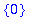Function: gmax - find floating-point approximations to the maximum of an expression on a real interval.

Calling sequence:

gmax( expr, x = a .. b);
gmax( expr, x = a .. b, 'x0');

Parameters:

expr - the expression, involving one variable

x - the variable (a name)

a, b - endpoints of the interval (real constants).

x0 - (optional) a name to use for saving the set of x values at which the maximum is attained.

Description:

gmax computes numerically the maximum value of an expression expr in one variable x on the real interval a .. b (including endpoints).

If the optional third argument is included, it must be a name. It will be assigned the set of x values at which the maximum is attained. Using quotes ( 'x0' ) to delay evaluation ensures that this will work even if x0 has previously been assigned a value.

Only one variable is allowed: the expression must evaluate to a real constant when any constant value in the interval a .. b is substituted for x .

The expression and all subexpressions should have at least two continuous derivatives on the interval. In particular, infinite limits at the endpoints, or indeterminate forms (such as f/g where f and g both approach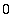or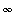at the endpoints) may cause trouble.

An exception to the requirements of continuity and differentiability is in the case of an expression defined piecewise, using piecewise , signum , Heaviside , abs , min or max , as long as convert(...,pwlist) can convert it to a list of expressions on different intervals. If this can't be done, an error occurs.

Infinite endpoints are allowed, but are not likely to work unless the limits of the expression at those endpoints are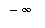or finite.

Since numerical techniques are used, the accuracy of the results is limited. In particular, a maximum where the second and third derivatives of the expression are 0 may be hard to locate (the maximum value should be accurate, but the location of the maximum may not be). Increasing Digits should also improve accuracy. Also, if the maximum value is attained at several points, roundoff error may prevent gmax from recognizing that the values at these points are the same.

In some difficult cases gmax may take a very long time. In particular, this will happen if the function is complicated or changes direction rapidly in the interval.

gmax uses evalr to do interval arithmetic, and is therefore subject to the weaknesses of that procedure. In particular, it doesn't work with the two-variable version of arctan .

This function is part of the Maple Advisor Database library, and must be loaded before use by the command readlib(gmin); .

Examples:

> gmax( sin(x) +x -x^2/2 , x = -1 .. 1 );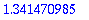> gmax( sin(x) + x -x^2/2 , x = -1 .. 1, 'x0');> x0;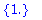> gmax(sin(x) + x - x^2/2, x = -infinity .. infinity,'x0');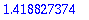> x0;> gmax(x^2-x^4,x=-2..2,'x0');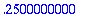> x0;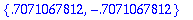> gmax(1/x,x=0..infinity,'x0');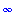> x0;